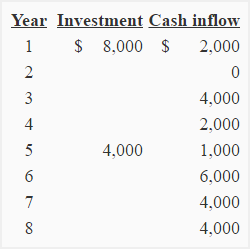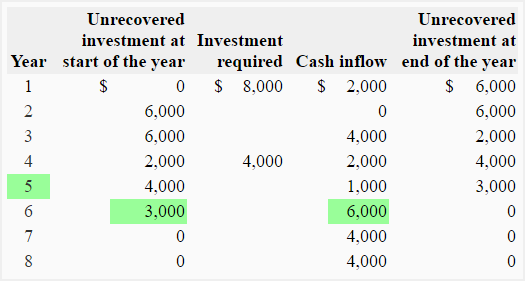# Exercise-8 (Computation of payback period – uneven cash flows)

The investment and expected cash inflows of a project over 8-year period is given below:Required: Compute the payback period of the project. Would the project be acceptable if the maximum desired payback period is 7 years?

## Solution:

As the expected cash flows is uneven (different cash flows in different periods), the payback formula cannot be used to compute payback period of this project. The payback period for this project would be computed by tracking the unrecovered investment year by year.Payback period = years before full recovery + (Unrecovered investment at start of the year/Cash flow during the year)

= 5 + (3,000/6,000)

= 5 + 0.5

= 5.5 years or 5 years and *6 months

*0.5 × 12

The entire investment is expected to recover by the middle of sixth year. The payback period of this project is, therefore, 5.5 years or 5 years and six moths.

Conclusion:

The project is acceptable because payback period promised by the project is shorter than the maximum desired payback period of the management.

A D V E R T I S E M E N T
15 Comments on Exercise-8 (Computation of payback period – uneven cash flows)
1.Perfect solution for my problem. Thank you for this work.

2.sabirali

Thanks, nice solution.

1.Accounting for Management

Welcome Sabirali.

3.jony

how to calculate month and day in payback period?

4.willie

I don’t understand the solution to this problem, need help.

5.priya

it was very useful

6.priya

need some more problems to practise

7.Accounting For Management

@Priya
OK, will include.

8.willie

pls explain better

9.Richard Branson

You know that the money is paid back during the 6th year, but to work out the month you do this.

(Unrecovered Investment at the Beginning of the year/Cash Inflow for that year) x 12 months

So, if you look back at the table you can find these figures and put it into the equation (don’t worry about minus figures).

(3000/6000)x 12 months = 6 months

Therefore, the answer will be 6 years and 6 months.
Understand?

10.mumin anelka

11.Yousuf

Can anybody help me to calculate the ROI/IRR/PBP on investment of non revenue items

Like : Kitchen stove heavy duty

12.Aasha

Good work… Must appreciate

13.Azam

if negative cash flows in first two years then how to use payback sheet

14.ESKAHUN

How to calculate Unrecovered Investment at the start and end year